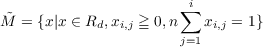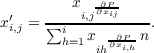#### Vol. 43, No. 3, 1972

 Download this articleFor screen For printingRecent Issues Vol. 325: 1  2 Vol. 324: 1  2 Vol. 323: 1  2 Vol. 322: 1  2 Vol. 321: 1  2 Vol. 320: 1  2 Vol. 319: 1  2 Vol. 318: 1  2Online Archive Volume: Issue:The Journal Subscriptions Editorial Board Officers Contacts Submission Guidelines Submission Form Policies for Authors ISSN: 1945-5844 (e-only) ISSN: 0030-8730 (print) Special Issues Author Index To Appear Other MSP Journals
Invariant functions of an iterative process for maximization of a polynomial

### Peter F. Stebe

Vol. 43 (1972), No. 3, 765–783
##### Abstract

Let P be a polynomial with real non-negative coefficients and variables xij,i = 1,,k,j = 1,,ni. Let d = k1ni. Let Rd be the d-dimensional real vector space. Let M be the subset of Rd defined bywhere the symbols xi,j denote the components of x. If x is a vector in the interior of M, define τ(x) as the vector in M with components xi,jgiven byThe expression on the right is evaluated at x. The transformation τ is defined on the boundary of M by the same formula if the denominators do not vanish.

Let F be the set of fixed points of τ in M. It is shown that if τ is a homeomorphism of M onto itself, there is a set of dk functions f1, , fdk defined on MF such that fi(x) = fi(τ(x)) for x MF. The functions fi are continuous and independent on an open dense subset of MF. Explicit expressions for certain invariant functions are also obtained.

Primary: 58E15
Secondary: 54H15
##### Milestones